# WHOLESALE CUSTOMERS DATASET

`df = pd.read_csv("/content/Wholesale customers data.csv")`

`print(df)`
`normalized_X = preprocessing.normalize(X)`
`X_train, X_test, y_train, y_test = train_test_split(X, y, test_size=0.2)`

# CART (Classification And Regression Trees)

`import pandas as pdfrom sklearn.tree import DecisionTreeClassifier from sklearn.model_selection import train_test_splitfrom sklearn import metrics`
`clf = DecisionTreeClassifier() clf = clf.fit(X_train,y_train)  y_pred = clf.predict(X_test)`
`print("Accuracy:",metrics.accuracy_score(y_test, y_pred))`
`from sklearn.tree import export_graphvizfrom sklearn.externals.six import StringIO  from IPython.display import Image  import pydotplusdot_data = StringIO()export_graphviz(clf, out_file=dot_data,                  filled=True, rounded=True,                special_characters=True)graph = pydotplus.graph_from_dot_data(dot_data.getvalue())  graph.write_png('wines.png')Image(graph.create_png())`

# Logistic Regression

`from sklearn.linear_model import LogisticRegressionfrom sklearn import metrics`
`logreg = LogisticRegression()logreg.fit(X_train, y_train)`
`y_pred = logreg.predict(X_test)print('Accuracy of logistic regression classifier on test set: {:.2f}'.format(logreg.score(X_test, y_test)))`
`from sklearn.metrics import classification_reportprint(classification_report(y_test, y_pred))`

# Perceptron

`pn = Perceptron(tol=1e-3, random_state=0)pn.fit(X_train, y_train)`
`pn.score(X_train,y_train)`
`pn.score(X_test,y_test)`

# Neural Network

`from keras.models import Sequentialfrom keras.layers import Densefrom keras.utils import to_categorical`
`model = Sequential()model.add(Dense(13, input_dim=13, activation='relu'))model.add(Dense(7, activation='relu'))model.add(Dense(4, activation='softmax'))model.compile(loss='binary_crossentropy', optimizer='adam', metrics=['accuracy'])`
`y_test_cat=to_categorical(y_test)y_train_cat=to_categorical(y_train)model.fit(X_train, y_train_cat, epochs=150, batch_size=10)`
`_, accuracy = model.evaluate(X_train, y_train_cat)print('Accuracy: %.2f' % (accuracy*100))`
`_, accuracy = model.evaluate(X_test, y_test_cat)print('Accuracy: %.2f' % (accuracy*100))`

# Random Forest

`from sklearn.ensemble import RandomForestClassifierclassifier = RandomForestClassifier(n_estimators = 900, criterion = 'gini', random_state = 0)classifier.fit(X_train, y_train)y_pred = classifier.predict(X_test)`
`print(classifier.score(X_test, y_test)`

--

--

--

## More from Sriraag Av

Love podcasts or audiobooks? Learn on the go with our new app.

## Handwritten Digit Recognition made easier with Yonohub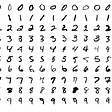## Serverless Deployment of fastai on Azure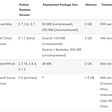## Introduction to Machine Learning## Edge Detection## Part-of-speech tag a string, filter to pronouns in Go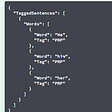## Evolutionary Reinforcement Learning in an Ecosystem Based Environment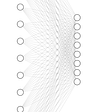## What are Isolation Forests?## Randomized Optimization Algorithm Comparison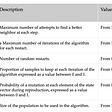## Walking Through Training Models## Evaluating classification models simplified.